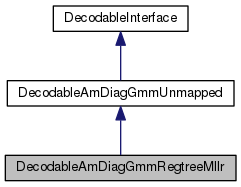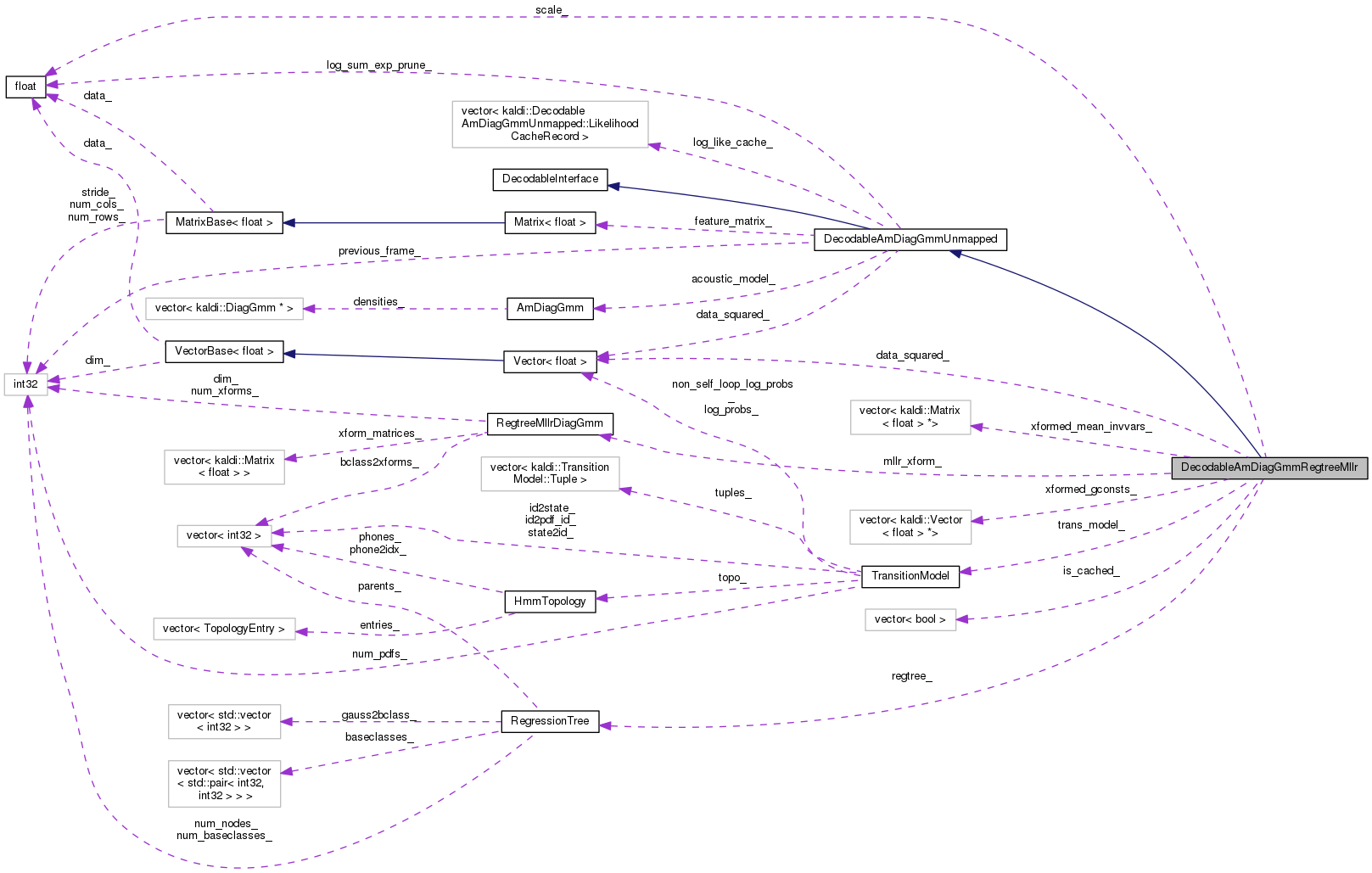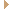DecodableAmDiagGmmRegtreeMllr Class Reference

`#include <decodable-am-diag-gmm-regtree.h>`

Inheritance diagram for DecodableAmDiagGmmRegtreeMllr:[legend]
Collaboration diagram for DecodableAmDiagGmmRegtreeMllr:[legend]

## Public Member Functions

DecodableAmDiagGmmRegtreeMllr (const AmDiagGmm &am, const TransitionModel &tm, const Matrix< BaseFloat > &feats, const RegtreeMllrDiagGmm &mllr_xform, const RegressionTree &regtree, BaseFloat scale, BaseFloat log_sum_exp_prune=-1.0)

~DecodableAmDiagGmmRegtreeMllr ()

virtual BaseFloat LogLikelihood (int32 frame, int32 tid)
Returns the log likelihood, which will be negated in the decoder. More...

virtual int32 NumFramesReady () const
The call NumFramesReady() will return the number of frames currently available for this decodable object. More...

virtual int32 NumIndices () const
Returns the number of states in the acoustic model (they will be indexed one-based, i.e. More...

const TransitionModelTransModel ()Public Member Functions inherited from DecodableAmDiagGmmUnmapped
DecodableAmDiagGmmUnmapped (const AmDiagGmm &am, const Matrix< BaseFloat > &feats, BaseFloat log_sum_exp_prune=-1.0)
If you set log_sum_exp_prune to a value greater than 0 it will prune in the LogSumExp operation (larger = more exact); I suggest 5. More...

virtual bool IsLastFrame (int32 frame) const
Returns true if this is the last frame. More...Public Member Functions inherited from DecodableInterface
virtual ~DecodableInterface ()

## Protected Member Functions

virtual BaseFloat LogLikelihoodZeroBased (int32 frame, int32 state_index)Protected Member Functions inherited from DecodableAmDiagGmmUnmapped
void ResetLogLikeCache ()

## Private Member Functions

void InitCache ()
Initializes the mean & gconst caches. More...

const Matrix< BaseFloat > & GetXformedMeanInvVars (int32 state_index)
Get the transformed means times inverse variances for a given pdf, and cache them. More...

const Vector< BaseFloat > & GetXformedGconsts (int32 state_index)
Get the cached (while computing transformed means) gconsts for likelihood calculation. More...

KALDI_DISALLOW_COPY_AND_ASSIGN (DecodableAmDiagGmmRegtreeMllr)

## Private Attributes

const TransitionModeltrans_model_

BaseFloat scale_

const RegtreeMllrDiagGmmmllr_xform_

const RegressionTreeregtree_

std::vector< Matrix< BaseFloat > *> xformed_mean_invvars_
Cache of transformed means time inverse variances for each state. More...

std::vector< Vector< BaseFloat > *> xformed_gconsts_
Cache of transformed gconsts for each state. More...

std::vector< boolis_cached_
Boolean variable per state to indicate whether the transformed means for that state are cached. More...

Vector< BaseFloatdata_squared_
Cached for fast likelihood calculation. More...

## Additional Inherited MembersProtected Attributes inherited from DecodableAmDiagGmmUnmapped
const AmDiagGmmacoustic_model_

const Matrix< BaseFloat > & feature_matrix_

int32 previous_frame_

BaseFloat log_sum_exp_prune_

std::vector< LikelihoodCacheRecordlog_like_cache_

## Detailed Description

Definition at line 80 of file decodable-am-diag-gmm-regtree.h.

## ◆ DecodableAmDiagGmmRegtreeMllr()

 DecodableAmDiagGmmRegtreeMllr ( const AmDiagGmm & am, const TransitionModel & tm, const Matrix< BaseFloat > & feats, const RegtreeMllrDiagGmm & mllr_xform, const RegressionTree & regtree, BaseFloat scale, BaseFloat log_sum_exp_prune = `-1.0` )
inline

Definition at line 82 of file decodable-am-diag-gmm-regtree.h.

88  :
89  DecodableAmDiagGmmUnmapped(am, feats, log_sum_exp_prune),
90  trans_model_(tm), scale_(scale), mllr_xform_(mllr_xform),
91  regtree_(regtree), data_squared_(feats.NumCols()) { InitCache(); }
void InitCache()
Initializes the mean & gconst caches.
MatrixIndexT NumCols() const
Returns number of columns (or zero for empty matrix).
Definition: kaldi-matrix.h:67
Vector< BaseFloat > data_squared_
Cached for fast likelihood calculation.
DecodableAmDiagGmmUnmapped(const AmDiagGmm &am, const Matrix< BaseFloat > &feats, BaseFloat log_sum_exp_prune=-1.0)
If you set log_sum_exp_prune to a value greater than 0 it will prune in the LogSumExp operation (larg...

## ◆ ~DecodableAmDiagGmmRegtreeMllr()

 ~DecodableAmDiagGmmRegtreeMllr ( )

Definition at line 91 of file decodable-am-diag-gmm-regtree.cc.

References kaldi::DeletePointers().

91  {
94 }
void DeletePointers(std::vector< A *> *v)
Deletes any non-NULL pointers in the vector v, and sets the corresponding entries of v to NULL...
Definition: stl-utils.h:184
std::vector< Vector< BaseFloat > *> xformed_gconsts_
Cache of transformed gconsts for each state.
std::vector< Matrix< BaseFloat > *> xformed_mean_invvars_
Cache of transformed means time inverse variances for each state.

## ◆ GetXformedGconsts()

 const Vector< BaseFloat > & GetXformedGconsts ( int32 state_index )
private

Get the cached (while computing transformed means) gconsts for likelihood calculation.

The 'state_index' is 0-based.

Definition at line 180 of file decodable-am-diag-gmm-regtree.cc.

References KALDI_ASSERT, and KALDI_ERR.

181  {
182  if (!is_cached_[state]) {
183  KALDI_ERR << "GConsts not cached for state: " << state << ". Must call "
184  << "GetXformedMeanInvVars() first.";
185  }
186  KALDI_ASSERT(xformed_gconsts_[state] != NULL);
187  return *xformed_gconsts_[state];
188 }
std::vector< Vector< BaseFloat > *> xformed_gconsts_
Cache of transformed gconsts for each state.
#define KALDI_ERR
Definition: kaldi-error.h:147
#define KALDI_ASSERT(cond)
Definition: kaldi-error.h:185
std::vector< bool > is_cached_
Boolean variable per state to indicate whether the transformed means for that state are cached...

## ◆ GetXformedMeanInvVars()

 const Matrix< BaseFloat > & GetXformedMeanInvVars ( int32 state_index )
private

Get the transformed means times inverse variances for a given pdf, and cache them.

The 'state_index' is 0-based.

Definition at line 151 of file decodable-am-diag-gmm-regtree.cc.

152  {
153  if (is_cached_[state]) { // found in cache
154  KALDI_ASSERT(xformed_mean_invvars_[state] != NULL);
155  KALDI_VLOG(3) << "For PDF index " << state << ": transformed means "
156  << "found in cache.";
157  return *xformed_mean_invvars_[state];
158  } else { // transform the means and cache them
159  KALDI_ASSERT(xformed_mean_invvars_[state] == NULL);
160  KALDI_VLOG(3) << "For PDF index " << state << ": transforming means.";
161  int32 num_gauss = acoustic_model_.GetPdf(state).NumGauss(),
162  dim = acoustic_model_.Dim();
163  const Vector<BaseFloat> &weights = acoustic_model_.GetPdf(state).weights();
164  const Matrix<BaseFloat> &invvars = acoustic_model_.GetPdf(state).inv_vars();
165  xformed_mean_invvars_[state] = new Matrix<BaseFloat>(num_gauss, dim);
167  xformed_mean_invvars_[state]);
168  xformed_gconsts_[state] = new Vector<BaseFloat>(num_gauss);
169  // At this point, the transformed means haven't been multiplied with
170  // the inv vars, and they are used to compute gconsts first.
171  ComputeGconsts(weights, *xformed_mean_invvars_[state], invvars,
172  xformed_gconsts_[state]);
173  // Finally, multiply the transformed means with the inv vars.
174  xformed_mean_invvars_[state]->MulElements(invvars);
175  is_cached_[state] = true;
176  return *xformed_mean_invvars_[state];
177  }
178 }
kaldi::int32 int32
std::vector< Vector< BaseFloat > *> xformed_gconsts_
Cache of transformed gconsts for each state.
std::vector< Matrix< BaseFloat > *> xformed_mean_invvars_
Cache of transformed means time inverse variances for each state.
const Vector< BaseFloat > & weights() const
Definition: diag-gmm.h:178
int32 NumGauss() const
Returns the number of mixture components in the GMM.
Definition: diag-gmm.h:72
int32 Dim() const
Definition: am-diag-gmm.h:79
void GetTransformedMeans(const RegressionTree &regtree, const AmDiagGmm &am, int32 pdf_index, MatrixBase< BaseFloat > *out) const
Get all the transformed means for a given pdf.
DiagGmm & GetPdf(int32 pdf_index)
Accessors.
Definition: am-diag-gmm.h:119
#define KALDI_ASSERT(cond)
Definition: kaldi-error.h:185
#define KALDI_VLOG(v)
Definition: kaldi-error.h:156
std::vector< bool > is_cached_
Boolean variable per state to indicate whether the transformed means for that state are cached...
static void ComputeGconsts(const VectorBase< BaseFloat > &weights, const MatrixBase< BaseFloat > &means, const MatrixBase< BaseFloat > &inv_vars, VectorBase< BaseFloat > *gconsts_out)
const Matrix< BaseFloat > & inv_vars() const
Definition: diag-gmm.h:180

## ◆ InitCache()

 void InitCache ( )
private

Initializes the mean & gconst caches.

Definition at line 97 of file decodable-am-diag-gmm-regtree.cc.

97  {
98  if (xformed_mean_invvars_.size() != 0)
100  if (xformed_gconsts_.size() != 0)
102  int32 num_pdfs = acoustic_model_.NumPdfs();
103  xformed_mean_invvars_.resize(num_pdfs);
104  xformed_gconsts_.resize(num_pdfs);
105  is_cached_.resize(num_pdfs, false);
107 }
void DeletePointers(std::vector< A *> *v)
Deletes any non-NULL pointers in the vector v, and sets the corresponding entries of v to NULL...
Definition: stl-utils.h:184
kaldi::int32 int32
std::vector< Vector< BaseFloat > *> xformed_gconsts_
Cache of transformed gconsts for each state.
std::vector< Matrix< BaseFloat > *> xformed_mean_invvars_
Cache of transformed means time inverse variances for each state.
int32 NumPdfs() const
Definition: am-diag-gmm.h:82
std::vector< bool > is_cached_
Boolean variable per state to indicate whether the transformed means for that state are cached...

## ◆ KALDI_DISALLOW_COPY_AND_ASSIGN()

 KALDI_DISALLOW_COPY_AND_ASSIGN ( DecodableAmDiagGmmRegtreeMllr )
private

## ◆ LogLikelihood()

 virtual BaseFloat LogLikelihood ( int32 frame, int32 index )
inlinevirtual

Returns the log likelihood, which will be negated in the decoder.

The "frame" starts from zero. You should verify that NumFramesReady() > frame before calling this.

Reimplemented from DecodableAmDiagGmmUnmapped.

Definition at line 95 of file decodable-am-diag-gmm-regtree.h.

95  {
96  return scale_*LogLikelihoodZeroBased(frame,
98  }
int32 TransitionIdToPdfFast(int32 trans_id) const
virtual BaseFloat LogLikelihoodZeroBased(int32 frame, int32 state_index)

## ◆ LogLikelihoodZeroBased()

 BaseFloat LogLikelihoodZeroBased ( int32 frame, int32 state_index )
protectedvirtual

Reimplemented from DecodableAmDiagGmmUnmapped.

Definition at line 190 of file decodable-am-diag-gmm-regtree.cc.

191  {
192 // KALDI_ERR << "Function not completely implemented yet.";
193  KALDI_ASSERT(frame < NumFramesReady() && frame >= 0);
194  KALDI_ASSERT(state < NumIndices() && state >= 0);
195
196  if (log_like_cache_[state].hit_time == frame) {
197  return log_like_cache_[state].log_like; // return cached value, if found
198  }
199
200  const DiagGmm &pdf = acoustic_model_.GetPdf(state);
201  const VectorBase<BaseFloat> &data = feature_matrix_.Row(frame);
202
203  // check if everything is in order
204  if (pdf.Dim() != data.Dim()) {
205  KALDI_ERR << "Dim mismatch: data dim = " << data.Dim()
206  << " vs. model dim = " << pdf.Dim();
207  }
208
209  if (frame != previous_frame_) { // cache the squared stats.
210  data_squared_.CopyFromVec(feature_matrix_.Row(frame));
211  data_squared_.ApplyPow(2.0);
212  previous_frame_ = frame;
213  }
214
215  const Matrix<BaseFloat> &means_invvars = GetXformedMeanInvVars(state);
216  const Vector<BaseFloat> &gconsts = GetXformedGconsts(state);
217
218  Vector<BaseFloat> loglikes(gconsts); // need to recreate for each pdf
219  // loglikes += means * inv(vars) * data.
220  loglikes.AddMatVec(1.0, means_invvars, kNoTrans, data, 1.0);
221  // loglikes += -0.5 * inv(vars) * data_sq.
222  loglikes.AddMatVec(-0.5, pdf.inv_vars(), kNoTrans, data_squared_, 1.0);
223
224  BaseFloat log_sum = loglikes.LogSumExp(log_sum_exp_prune_);
225  if (KALDI_ISNAN(log_sum) || KALDI_ISINF(log_sum))
226  KALDI_ERR << "Invalid answer (overflow or invalid variances/features?)";
227
228  log_like_cache_[state].log_like = log_sum;
229  log_like_cache_[state].hit_time = frame;
230
231  return log_sum;
232 }
virtual int32 NumIndices() const
Returns the number of states in the acoustic model (they will be indexed one-based, i.e.
#define KALDI_ISINF
Definition: kaldi-math.h:73
Vector< BaseFloat > data_squared_
Cached for fast likelihood calculation.
float BaseFloat
Definition: kaldi-types.h:29
const SubVector< Real > Row(MatrixIndexT i) const
Return specific row of matrix [const].
Definition: kaldi-matrix.h:188
const Matrix< BaseFloat > & GetXformedMeanInvVars(int32 state_index)
Get the transformed means times inverse variances for a given pdf, and cache them.
#define KALDI_ERR
Definition: kaldi-error.h:147
const Matrix< BaseFloat > & feature_matrix_
virtual int32 NumFramesReady() const
The call NumFramesReady() will return the number of frames currently available for this decodable obj...
std::vector< LikelihoodCacheRecord > log_like_cache_
DiagGmm & GetPdf(int32 pdf_index)
Accessors.
Definition: am-diag-gmm.h:119
#define KALDI_ISNAN
Definition: kaldi-math.h:72
#define KALDI_ASSERT(cond)
Definition: kaldi-error.h:185
const Vector< BaseFloat > & GetXformedGconsts(int32 state_index)
Get the cached (while computing transformed means) gconsts for likelihood calculation.

 virtual int32 NumFramesReady ( ) const
inlinevirtual

The call NumFramesReady() will return the number of frames currently available for this decodable object.

This is for use in setups where you don't want the decoder to block while waiting for input. This is newly added as of Jan 2014, and I hope, going forward, to rely on this mechanism more than IsLastFrame to know when to stop decoding.

Reimplemented from DecodableAmDiagGmmUnmapped.

Definition at line 100 of file decodable-am-diag-gmm-regtree.h.

Referenced by main().

100 { return feature_matrix_.NumRows(); }
const Matrix< BaseFloat > & feature_matrix_
MatrixIndexT NumRows() const
Returns number of rows (or zero for empty matrix).
Definition: kaldi-matrix.h:64

## ◆ NumIndices()

 virtual int32 NumIndices ( ) const
inlinevirtual

Returns the number of states in the acoustic model (they will be indexed one-based, i.e.

from 1 to NumIndices(); this is for compatibility with OpenFst).

Reimplemented from DecodableAmDiagGmmUnmapped.

Definition at line 103 of file decodable-am-diag-gmm-regtree.h.

103 { return trans_model_.NumTransitionIds(); }
int32 NumTransitionIds() const
Returns the total number of transition-ids (note, these are one-based).

## ◆ TransModel()

 const TransitionModel* TransModel ( )
inline

Definition at line 105 of file decodable-am-diag-gmm-regtree.h.

105 { return &trans_model_; }

## ◆ data_squared_

 Vector data_squared_
private

Cached for fast likelihood calculation.

Definition at line 134 of file decodable-am-diag-gmm-regtree.h.

## ◆ is_cached_

 std::vector is_cached_
private

Boolean variable per state to indicate whether the transformed means for that state are cached.

Definition at line 132 of file decodable-am-diag-gmm-regtree.h.

## ◆ mllr_xform_

 const RegtreeMllrDiagGmm& mllr_xform_
private

Definition at line 122 of file decodable-am-diag-gmm-regtree.h.

## ◆ regtree_

 const RegressionTree& regtree_
private

Definition at line 123 of file decodable-am-diag-gmm-regtree.h.

## ◆ scale_

 BaseFloat scale_
private

Definition at line 121 of file decodable-am-diag-gmm-regtree.h.

## ◆ trans_model_

 const TransitionModel& trans_model_
private

Definition at line 120 of file decodable-am-diag-gmm-regtree.h.

## ◆ xformed_gconsts_

 std::vector< Vector* > xformed_gconsts_
private

Cache of transformed gconsts for each state.

Definition at line 129 of file decodable-am-diag-gmm-regtree.h.

## ◆ xformed_mean_invvars_

 std::vector< Matrix* > xformed_mean_invvars_
private

Cache of transformed means time inverse variances for each state.

Definition at line 127 of file decodable-am-diag-gmm-regtree.h.

The documentation for this class was generated from the following files: